## August 1, 2013

### Spans and the Categorified Heisenberg Algebra

#### Posted by John BaezThere’s a nice conference coming up soon in the wild west of China:

• Higher Structures in China IV, Lanzhou University, August 14-16, 2013, organized by Li Guo, Yanfeng Luo, Weiwei Pan, Yunhe Sheng and Chenchang Zhu.

Chongying Dong is giving a short course on quantum dimensions for vertex operator algebra representations, and quantum Galois theory. Pierre-Louis Curien will give one on higher-dimensional rewriting and applications to coherent presentations of Artin groups. And I’ll give one about spans of groupoids and the combinatorics underlying the key relation in the Heisenberg algebra:

$a a^\dagger = a^\dagger a + 1$

Besides talking about these topics, which form a nice example of categorified algebra, I thought this would be a good chance to familiarize more people with the second column of the periodic table:

• bicategories
• monoidal bicategories
• braided monoidal bicategories
• sylleptic monoidal bicategories
• symmetric monoidal bicategories

So, I’ll use Mike Stay’s wonderful diagrams to explain these things. You can see my slides here.

Heisenberg reinvented matrices while discovering quantum mechanics, and the algebra generated by annihilation and creation operators $a$ and $a^\dagger$ obeying

$a a^\dagger = a^\dagger a + 1$

was named after him: the Heisenberg algebra. It turns out that matrices arise naturally from ‘spans’, where a span between two objects is just a third object with maps to both those two: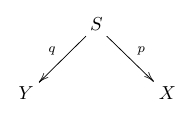Spans also let you formalize the simple combinatorial interpretation of the equation $a a^\dagger = a^\dagger a + 1$, namely,

there’s 1 more way to add an element to a set and then remove one than to remove one and then add one.

More recently, Mikhail Khovanov introduced a ‘categorified’ Heisenberg algebra, where this equation holds only up to isomorphism, and this isomorphism obeys new relations of its own.

The meaning of these new relations was initially rather mysterious, at least to me. However, Jeffrey Morton and Jamie Vicary have shown that these, too, have a nice interpretation in terms of spans. We can begin to formalize this using the work of Alex Hoffnung and Mike Stay, who have shown that spans of groupoids are morphisms in a symmetric monoidal bicategory.

Here are the slides:

Part 1 – How Jeffrey Morton and Jamie Vicary categorified the Heisenberg algebra using spans of groupoids.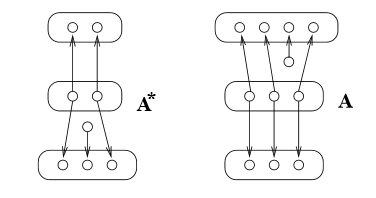Part 2 – The definition of symmetric monoidal bicategory, following Mike Stay.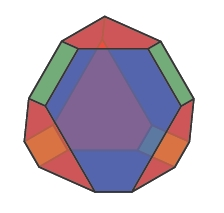Part 3 – How the categorified Heisenberg algebra shows up in the theory of Young diagrams and Schur functors.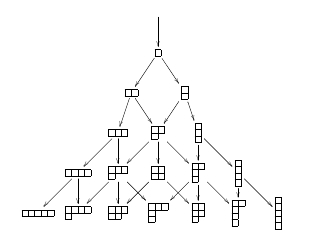For more on this subject try these papers:

For more on the physics of spans, try this other talk of mine: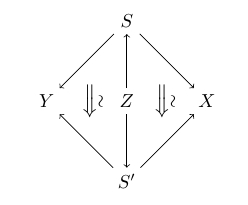The pictures of creation and annihilation spans above are by Jeffrey Morton. The truncated shuffle polytope, a coherence law in the definition of braided monoidal bicategory, was drawn by Mike Stay. The Young diagrams with arrows showing the action of the categorified creation operator were drawn by Jeffrey Morton and Jamie Vicary.

Posted at August 1, 2013 3:04 AM UTC

TrackBack URL for this Entry:   https://golem.ph.utexas.edu/cgi-bin/MT-3.0/dxy-tb.fcgi/2641

### Re: Spans and the Categorified Heisenberg Algebra

Posted by: Allen K. on August 1, 2013 4:21 AM | Permalink | Reply to this

### Re: Spans and the Categorified Heisenberg Algebra

Thanks, fixed! I left out the

http://math.ucr.edu/home/baez/spans/

on these two when copying the links from my own page (relative links) to here (absolute ones).

Posted by: John Baez on August 1, 2013 5:04 AM | Permalink | Reply to this

### Re: Spans and the Categorified Heisenberg Algebra

Over on G+, people started talking about this picture of the creation operator acting on the category of Schur functors:Owen Maresh asked “is it useful to think of that as a Pascal’s Triangle like-object?﻿” Somewhat to my surprise, Layra Idarani said the answer is yes!

We can count the dimension of a symmetric group representation labelled by one of the Young diagrams here by counting the number of paths from the top of the picture to that diagram.

So, to get the dimension of each representation, you just add up the dimensions of the representations that have arrows pointing to it! (You start off with dimension 1 for the Young diagram with no boxes.)

In more detail:

There is a Pascal-ish behavior for the hook numbers associated to the Young diagrams. For a box $b$ in a diagram, we find the hook length of $b$, which is just the number of boxes to the right of $b$ in the same row, plus the number of boxes below $b$ in the same column, plus 1 for $b$ itself. Then for a diagram $D$ with $n$ boxes in it, we define $H(D)$ to be $n!$ divided by the product of all the hook length for all the boxes in the diagram.

Going back to the picture above, if you write out the hook numbers for each of the Young diagrams, you’ll find that $H(D)$ is just the sum of the $H(D_i)$ for $D_i$ contained in D and having one fewer box.

So for instance,

■■■
■■

has a hook number of $6!/(5*3*1*3*1*1) = 16$, and contains

■■■
■■

with hook number $5!/(4*3*1*2*1) = 5$,

■■■

with hook number $5!/(5*2*1*2*1) = 6$, and

■■
■■

with hook number $5!/(4*2*3*1*1) = 5$

This is just the decategorification of the usual branching rules for representations of the symmetric groups, where a diagram $D$ with n boxes corresponds to a vector space $V(D)$ with dimension $H(D)$ equipped with an action of $S_n$, and restricting to an action of $S_{n-1}$ gives a decomposition of $V(D)$ into the representations of $S_{n-1}$ whose diagrams are contained in D, each with multiplicity 1.

Of course, unlike with Pascal’s triangle, as you increase the number of boxes, diagrams start to have more and more sub-diagrams so the addition gets a little hairy.

I should add that the annihilation operator on the category of Schur functors, described on my talk, simply amounts to a functor sending each representation of $S_n$ (for any $n$) to its restriction to $S_{n-1}$. The creation operator, shown in the picture above, is the adjoint functor. So, it does ‘induction’ to send representations of $S_{n-1}$ to representations of $S_n$.

Posted by: John Baez on August 5, 2013 5:05 AM | Permalink | Reply to this

### Re: Spans and the Categorified Heisenberg Algebra

What’s the simplest category whose category of spans is (dagger compact) equivalent to FdHilb?

Posted by: Mark Gomer on August 7, 2013 9:58 PM | Permalink | Reply to this

### Re: Spans and the Categorified Heisenberg Algebra

I haven’t proved it, but I don’t believe any category has a category of spans that’s equivalent to FDHilb (the category of finite-dimensional Hilbert spaces and linear maps). At least I don’t know such a category. Do you one?

If you take the category of finite sets, and form the category of (isomorphism classes of) spans in that, you get a category Span(FinSet). This maps into FDHilb in an obvious way: a span of finite sets gives a matrix of natural numbers, which is a special case of a matrix of complex numbers, which gives a morphism in FDHilb.

You can add spans of finite sets; this corresponds to adding matrices. This makes Span(FinSet) into a category enriched in finitely generated free commutative monoids. There is monoidal functor from FinFreeCommMon to FDHilb that maps the finitely generated free commutative monoid $\mathbb{N}^k$ to the finite-dimensional Hilbert space $\mathbb{C}^k$. Applying this ‘change of base’ to Span(FinSet) we get a category enriched in FDHilb, and this is equivalent to FDHilb.

That’s the easiest way I know to get FDHilb from a category of spans.

Posted by: John Baez on August 8, 2013 10:47 AM | Permalink | Reply to this

Post a New Comment